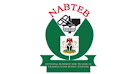## Ads Top

Chemistry Paper II Practical AnswersFriday 12/05/2017 - 9:00a.m-11:00a.m

ANSWERS LOADING....

1a) Volume of pipette used =25cm^3
Indicator used = Methyl Orange
Table of observation
Burette Reading | Rough | 1st | 2nd |3rd
Final Reading|24.50 |23.50 |23.40 |23.60
Initial Reading (Cm^3)| 0.00| 0.00| 0.00| 0.00
Volume of Acid Used|24.50| 23.50| 23.40| 23.60
Average volume of acid used =1st + 2nd + 3rd/3
=23.50 + 23.40 + 23.60/3
=70.5/3 = 23.50cm^3
1b) Concentration of solution B in Mol/dm^3
CAVA/CBVB=nA/nB
CA= 0.03mol/dm^3, VA=23.50cm^3, nA=2, nB=1, VB= 25cm^3, CB= ?
CB= CAVAnB/VBnA
CB= 0.03 x 23.5 x 1/25 x 2
=0.705/50 =0.0141
Concentration of Solution B in mol/dm^3=0.0141mol/dm^3
1bii) Molar mass of Nacl
=23 + 35.5 = 58.5g/mol
Na2Co3 in 1dm^3 of B = Molar Conc x Volume
=0.0141 x 1
=0.0141mole
From the equation
Na2Co3 + 2Hcl——>2Nacl + H2O + Co2
1mole of Na2Co3 produces 1mole of Nacl
0.0141mole of Na2Co3 librates
2 x 0.0141mol of Nacl
=0.0282mol
Mass of Nacl =0.0282 x 58.5
=1.6497g
=1.65g
1biii) From the equation:
1mole of Na2Co3 produces 1mole of Co2 at S. T. P
1mole of Na2Co3 produces 22.4dm^3 of Co2 at S.T.P
Therefore, 0.0141mole of Na2Co3 will produce 22.4 x 0.0141
Volume of Co2=0.316dm^3
1bix) Molar Mass of H2x=(2 x 1) + X=(2 + X)g/mol
==================================
2) TEST | OBSERVATION | INFERENCE
a) C+ water mixed thoroughly and filtered | Partially Soluble in water with colourless filtrate and white residue | C is a mixture of soluble and insoluble salts
bi) Filtrate + NaOH(aq) in drops, then in excess | White precipitate is formed. The precipitate soluble in excess NaOH(aq)| Pb^2+, Zn^2+ or Al^3+ present
bii) Filtrate of NH3(aq) in drops, then in excess | White is formed. The precipitate is insoluble in excess NH3(aq)| Pb^2+ or Al^3+ present
biii) Filtrate + KI(aq) + warm and allow to cool| Yellow precipitate is formed. The precipitate reappears after cooling| Pb^2+ confirmed
c) Residue + HCL(aq)| The residue dissolves ,librating a colourless gas which turns lime water milky| Gas is Co2 from Co3^2-is present
d) Residue + NH3(aq) in drops, then in excess | White gelatinous precipitate is formed. The precipitate is soluble in excess NH3(aq)| Zn^2+ confirmed
==================================
3a)
NH4CL and NaN03 dissolves readity in cold water
3aii)
CuCO3 have a black residue on heating
3bi)
E=Cu0
3bii)
blue precipitate is formed the precipitate dissolves in aqeous ammonia to give a deep blue solution
3biii)
G is water (H20)
3biv)
A dark brown precipitate is formed at the bottom of the test tube which shows that copper has been displaced by zinc from its solution

#### No comments:

These Comments are 100% Moderated. Please Bear With us as It may Take Some Time to Reply Back.